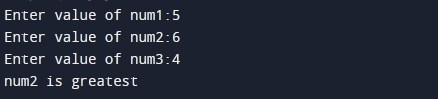# C Program to Find Greatest Number Among three Number

In this tutorial you will learn about the C Program to Find Greatest Number Among three Number and its application with practical example.

In this tutorial, we will learn to create a program to find greatest number among three numbers using C programming language. We will show you two different method to find greatest number among three numbers :

• Using C If statement
• Using Nested If else statement
• Using If else Ladder Statement

Prerequisites

Before starting with this tutorial we assume that you are best aware of the following C programming topics:

• C Data Types
• C Variables
• C Input Output
• C Operators
• C If else

## C Program to Find the Greatest Number Among Three Numbers Using If Statement

In this program we will compare three integer numbers and returns the greatest number as result. We would first declared and initialized the required variables. Next, we would prompt user to input three integer numbers to compare. Later in the program we will compare input numbers using C if Statement. Finally, we will display the largest number among three numbers.

Output:-In the above program, we have first declared and initialized a set variables required in the program.

• num1 = it holds the first number value
• num2 = it holds the second number value
• num3 = it holds the third number value

In the next statement user will be prompted to enter the three integer number values which will be assigned to variable ‘num1’ , ‘num2’ and ‘num3’ respectively. Then, we will perform the comparison of the given three numbers respectively.

num1 is greatest when following condition returns true:

num2 is greatest when following condition returns true:

num3 is greatest when following condition returns true:

Finally, we will display the largest number among three numbers using printf statement.

## C Program to Find the Greatest Number Among Three Numbers Using Nested If Else Statement

In this program we will compare three integer numbers and returns the greatest number as result. We would first declared and initialized the required variables. Next, we would prompt user to input three integer numbers to compare. Later in the program we will compare numbers using C nested if else Statement. Finally, we will display the largest number among three numbers.

Output:-In the above program, we have first declared and initialized a set variables required in the program.

• num1 = it holds the first number value
• num2 = it holds the second number value
• num3 = it holds the third number value

In the next statement user will be prompted to enter the three integer number values which will be assigned to variable ‘num1’ , ‘num2’ and ‘num3’ respectively. Then, we will perform the comparison of the given three numbers respectively. We have first if else statement to with following condition:

if it returns true, we have another if else statement:

if it return true, means  num1 is largest. If it return false, means num3 is largest.

If the outer if else statement returns false, then in the else block we have another if else statement:

if it return true, means  num2 is largest. If it return false, means num3 is largest.

## Find the Largest Number Among Three Numbers Using If else Ladder Statement

In this program we will compare three integer numbers and returns the greatest number as result. We would first declared and initialized the required variables. Next, we would prompt user to input three integer numbers to compare. Later in the program we will compare numbers using C if else ladder Statement. Finally, we will display the largest number among three numbers.

Output:-In the above program, we have first declared and initialized a set variables required in the program.

• num1 = it holds the first number value
• num2 = it holds the second number value
• num3 = it holds the third number value

In the next statement user will be prompted to enter the three integer number values which will be assigned to variable ‘num1’ , ‘num2’ and ‘num3’ respectively. Then, we will perform the comparison of the given three numbers respectively. We have first if else statement to with following condition:

if it return true, means  num1 is largest. If it return false, then we have else if statement:

if it return true, means  num2 is largest. If it return false, then we have final else statement that means num3 is largest.

In this tutorial we have learn about the C Program to Find Greatest Number Among three Number and its application with practical example. I hope you will like this tutorial.